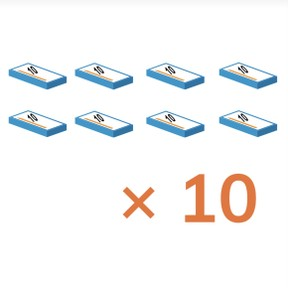Multiplying by 10

# Multiplying by 10

Students learn to multiply by 10.8,000 schools use Gynzy92,000 teachers use Gynzy1,600,000 students use Gynzy

## General

The students learn to multiply by 10.

## Standards

CCSS.Math.Content.3.NBT.A.3

## Learning objective

Students will be able to multiply with the number 10.

## Introduction

Have the students calculate how many objects there are. Have the students explain out loud how they reached their answer.

## Instruction

First explain that a multiplication problem is where you calculate that you have something a certain number of times. In the 10 times table you have another 10 each time you multiply. Show with help from the problem 56 x 1 and 56 x 10 that with a times 10 multiplication problem, the answer is also 10 times as much. Afterwards, show abstract problems and discuss that each time you have different numbers of groups of 10. It is useful to first calculate the problem in which you multiply the number by 1. Then you can easily calculate how much 10 times that amount is. The answer is 10 times as many. Have the students calculate several problems with the paint spots and explain that you have to think carefully about which problem the answer belongs to. Have the students practice with the problems, for which a number is missing. After this, explain how students can solve a story problem by following the steps. First they see what is being asked, then find the numbers they need, then state the problem and finally solve the problem. Practice this together with the students.

Check whether the students can multiply by 10 with the following questions:
- Can you give an example of 20, 10 times?
- What does the problem 16 × 10 mean?

## Quiz

The students test their understanding of multiplying by 10 through ten exercises. For some of the exercises they have a choice between possible answers, for some they must give an answer on their own, and other exercises are word problems.

## Closing

Discuss once again with the students that it is important to be able to multiply by 10, so that you can calculate how many you have if you have 10 a certain number of times. Ask how they can calculate a multiplication problem with 10 and have them solve a few problems with multiplying by 10. Then practice multiplying with 10 by sitting in a circle and throwing a ball, during which you name a multiplication problem with the number 10. The student catches the ball, states the answer, and throws it to the next student. Unless the forbidden number is in the answer. This is, to start, the number 2. Then you only throw the ball and do not answer. If you do give the answer, then you're out of the game. To make the game more difficult, you can discuss adding more than one forbidden number.

## Teaching tips

Make sure that students have a good understanding of multiplication problems by practicing this visually. To do this, allow students to use concrete materials, such as blocks, if they need to or write out the problem on a sheet of scrap paper. If students have difficulty with multiplying by 10, you can have them practice by counting in jumps of 10 and adding up each jump.

## Instruction materials

Ball and blocks

### The online teaching platform for interactive whiteboards and displays in schools

• Save time building lessons

• Manage the classroom more efficiently

• Increase student engagement